# Mikhailovsky and Levic: Entropy, Information and Complexity or Which Aims the Arrow of Time?

This below is my summery of a somewhat quirky article by George E. Mikhailovsky and Alexander P. Levic on MDPI. It suggests a mathematical model for the variation of complexity, using conditional local maximum entropy for (hierarchical) interrelated objects or elements in systems. I am not capable to verify whether this model makes sense mathematically. However I find the logic of it appealing because it brings a relation between entropy, information and complexity. I need this to be able to assess the complexity of my systems, i.e. businesses. Also it is based on / akin to ‘proven technology’ (i.e. existing models for these concepts in a mathematical grid) and it is seems to be more than a wild guess. Additionally it implicates relations between hierarchical levels and objects of a system, using a resources view. Lastly, and connecteed to this last issue, it addresses this ever-intriguing matter of irreversibility and the concept of time on different scales, and the mutual relation to time at a macroscopic level, i.e. how we experience it here and now.

This quote below from the last paragraph is a clue of why I find it important: “The increase of complexity, according to the general law of complification, leads to the achievement of a local maximum in the evolutionary landscape. This gets a system into a dead end where the material for further evolution is exhausted. Almost everybody is familiar with this, watching how excessive complexity (bureaucratization) of a business or public organization leads to the situation when it begins to serve itself and loses all potential for further development. The result can be either a bankruptcy due to a general economic crisis (external catastrophe) or, for example, self-destruction or decay into several businesses or organizations as a result of the loss of effective governance and, ultimately, competitiveness (internal catastrophe). However, dumping a system with such a local maximum, the catastrophe gives it the opportunity to continue the complification process and potentially achieve a higher peak.”

According to the second law entropy increases in isolated systems (Carnot, Clausius). Entropy is the first physical quantity that varies in time asymmetrically. The H-theorem of Ludwig Boltzmann shows how the irreversibility of entropy increase is derived from the reversibility of microscopic processes obeying Newtonian mechanics. He deduced the formula to:

(1) S = KblnW

S is entropy

Kb is the Boltzmann constant equal to 1.38×10 23 J/K

W is the number of microstates related to a given macrostate

This equation relates to values at different levels or scales in a system hierarchy, resulting in a irreversible parameter as a result.

In 1948, Shannon and Weaver (The Mathematical Theory of Communication) suggested a formula for informational entropy:

(2) H = −KΣpilog pi

K is an arbitrary positive constant

pi the probability of possible events

If we define the events as microstates, consider them equally probable and choose the nondimensional Boltzmann constant, the Shannon Equation (2) becomes the Boltzmann Equation (1). The Shannon equation is a generalisation of the Boltzmann equation with different probabilities for letters making up a message (different microstates leading to a macrostate of a system). Shannon says (p 50): “Quantities of the form H = −KΣpilog pi (the constant K merely amounts to a choice of a unit of measure) play a central role in information theory as measures of information, choice and uncertainty. The form of H will be recognized as that of entropy as defined in certain formulations of statistical mechanics, where pi is the probability of a system being in cell i of its phase space.”. Note that no reference is quoted to a difference between information and information entropy. Maximum entropy exists when probabilities in all locations, pi, are equal and the information of the system (message) is in maximum disorder. Relative entropy is the ratio of H to maximum entropy.

The meaning of these values has proven difficult, because the concept of entropy is generally seen as something negative, whereas the concept of information is seen as positive. This is an example by Mikhailovsky and Levic: “A crowd of thousands of American spectators at an international hockey match chants during the game “U-S-A! U-S-A!” We have an extremely ordered, extremely degenerated state with minimal entropy and information. Then, as soon as the period of the hockey game is over, everybody is starting to talk to each other during a break, and a clear slogan is replaced by a muffled roar, in which the “macroscopic” observer finds no meaning. However, for the “microscopic” observer who walks between seats around the arena, each separate conversation makes a lot of sense. If one writes down all of them, it would be a long series of volumes instead of three syllables endlessly repeated during the previous 20 minutes. As a result, chaos replaced order, the system degraded and its entropy sharply increased for the “macro-observer”, but for the “micro-observer” (and for the system itself in its entirety), information fantastically increased, and the system passed from an extremely degraded, simple, ordered and poor information state into a much more chaotic, complex, rich and informative one.” In summary: the level of orde depends on the observed level of hierarchy. Additionally, the value attributed to order has changed in time and so may have changed the qualification ‘bad’ and ‘good’ used for entropy and information respectively.

A third concept connected to order and chaos is complexity. The definition of algorithmic complexity K(x) of the final object x is the length of the shortest computer program that prints a full, but not excessive (i.e. minimal), binary description of x and then halts. The equation for Kolmogorov complexity is:

(3) K(x) = lpr + Min(lx)

D is a set of all possible descriptions dx in range x

L is the set of equipotent lengths lx of the descriptions dx in D

lpr is the binary length of the printing algorithm mentioned above

In case x is not binary, but some other description using n symbols, then:

(4) K(x) = lpr + Min((1/n)Σpi2log(pi))

Mikhailovsky and Levic conclude that, although Equation (4) for complexity is not

completely equivalent to Equations (1) and (2), it can be regarded as their generalization in a broader sense.

Now we define an abstract representation of the system as a category that combines a class of objects and a class of morphisms. Objects of the category explicate (nl: expliciteren) the system’s states and morphisms define admissible transitions from one state to another. Categories with the same objects, but differing morphisms are different and describe different systems. For example, a system with transformations as arbitrary conformities differs from a system where the same set of objects transforms only one-to-one. Processes taking place in the first system are richer than in the latter because the first allows transitions between states of a variable number of elements, while the second requires the same number of elements in different states.

Let us take a system described by category S and the system states X and A, identical to objects X and A from S. Invariant I {X in S} (A) is a number of morphisms from X to A in the category S preserving the structure of objects. In the language of systems theory, invariant I is a number of transformations of the state X into the state A, preserving the structure of the system. We interpret the structure of the system as its “macrostate”. Transformations of the state X into the state A will be interpreted as ways of obtaining the state A from state X, or as “microstates”. Then, the invariant of a state is the number of microstates preserving the macrostate of the system, which is consistent with the Boltzmann definition of entropy in Equation (1). More strictly: we determine generalized entropy of the state A of system S (relating to the state X of the same system) as a value:

(5) Hx (A) = ln( I{X in Q}(A) / I{X in Q}(A) )

I{X in Q}(A) is the number of morphisms from set X into set A in the category of structured sets Q, and I{X in Q}(A) is the number of morphisms from set X into set A in the category of structureless sets Q with the same cardinality (number of dimensions) as in category Q, but with an “erased structure”. In particular cases, generalized entropy has the usual “Boltzmann” or, if you like, “Shannon” look (example given). This represents a ratio of the number of transformations preserving the structure by the total number Q of transformations that can be interpreted as the probability of the formation of the state with a given structure. Statistical entropy (1), information (2) and algorithmic complexity (4) are only a few possible interpretations of Equation (5). It is important to emphasize that the formula for the generalized entropy is introduced with no statistic or probabilistic assumptions and is valid for any large or small amounts of elements of the system.

The amount of “consumed” (plus “lost”) resources determines “reading” of the so-called “metabolic clock” of the system. Construction of this metabolic clock implies the ability to count the number of elements replaced in the system. Therefore, a non-trivial application of the metabolic approach requires the ability to compare one structured set to another. This ability comes from a functorial method comparison of structures that offers system invariants as generalization of the concept “number of elements” for structureless sets. Note that the system that consumes several resources exists in several metabolic times. The entropy of the system is an “averager” of metabolic times, and entropy increases monotonically with the flow of each of metabolic time, i.e., entropy and metabolic times of a system are linked uniquely, monotonously and can be calculated one through the other. This relationship is given by:

(7)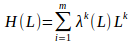Here, H is structural entropy, L ≡ {L1 , L2 , . ., Lm} the set of metabolic times (resources) of system and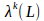Lagrange multipliers of the variational problem on the conditional maximum of structural entropy, restricted by flows of metabolic times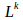. For the structure of sets with partitions where morphisms are preserving the partition mapping (or their dual compliances), the variational problem has the form:

(8)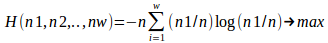It was proven that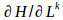≥ 0, i.e., structural entropy monotonously increases (or at least does not decrease) in the metabolic time of the system or entropy “production” does not decrease along a system’s trajectory in its state space (the theorem is analogous to the Boltzmann H-theorem for physical time). Such a relationship between generalized entropy and resourcescan be considered as a heuristic explanation of the origin of the logarithm in the dependence of entropy on the number of transformations: with logarithms the relationship between entropy and metabolic times becoming a power, not exponential, which in turn simplifies the formulas, which involve both parameterizations of time. Therefore, if the system metabolic time is, generally speaking, a multi-component magnitude and level-specific (relating to hierarchical levels of the system), then entropy time “averaging” metabolic times of the levels parameterizes system dynamics and returns the notion of the time to its usual universality.

The class of objects that explicates a system of categories can be presented as a system’s state space. An alternative to the postulation of the equations of motion in theoretical physics, biology, economy and other sciences is the postulation of extremal principles that generate variability laws of the systems studied. What needs to be extreme in a system? The category-functorial description gives a “natural” answer to this question, because category theory has a systematical method to compare system states. The possibility to compare the states by the strength of their structure allows one to offer an extremal principle for systems’ variation: from a given state, the system goes into a state having the strongest structure. According to the method, this function is the number of transformations admissible by structure of the system. However, a more usual formulation of the extremal principle can be obtained if we consider the monotonic function of the specific amount of admissible transformations that we defined as the generalized entropy of the state; namely given that the state of the system goes into a state for which the generalized entropy is maximal within the limits set by available resources. A generalized category-theoretic entropy allows not guessing and not postulating the objective functions, but strictly calculating them from the number of morphisms (transformations) allowed by the system structure.

Let us illustrate this with an example. Consider a very simple system consisting of a discrete space of 8 × 8 (like a chess board without dividing the fields on the black and white) and eight identical objects distributed arbitrary on these 64 elements of the space (cells). These objects can move freely from cell to cell, realizing two degrees of freedom each. The number of degrees of freedom of the system is twice as much as the number of objects due to the two-dimensionality of our space. We will consider the particular distribution of eight objects on 64 elements of our space (cells) as a system state that is equivalent in this case to a “microstate”. Thus, the number of possible states equals the number of combinations of eight objects from 64 ones: W8 = 64!/(64−8)!/8! = 4,426,165,368 .

Consider now more specific states when seven objects have arbitrary positions, while the position of the eighth one is completely determined by the positions of one, a few or all of the others. In this case, the number of degrees of freedom will reduce from 16 (eight by two) to 14 (seven by two), and the number of admissible states will decrease up to the number of combinations by seven objects, seven from 64 ones: W7 = 64!/(64−7)!/7! = 621,216,192

Let us name a set of these states a “macrostate”. Notice that the number of combinations of k elements from n calculated by the formula

(9) n! / (k! * (n-k)!)

is the cumulative number of “microstates” for “macrostates” with 16, 14, 12, and so on, degrees of freedom. Therefore, to reveal the number of “microstates” related exclusively to a given “macrostate”, we have to subtract W7 from W8 , W6 from W7, etc. These figures make quite clear that our simple model system being left to itself will inevitably move into a “macrostate” with more degrees of freedom and a larger number of admissible states, i.e., “microstates”. Two obvious conclusions immediately follow from these considerations:

• It is far more probable to find a system in a complex state than in a simple one.

• If a system came to a simple state, the probability that the next state will be simpler is immeasurably less than the probability that the next state will be more complicated.

This defines a practically irreversible increase of entropy, information and complexity, leading in turn to the irreversibility of time. For space 16 × 16, we could speak about practical irreversibility only, when reversibility is possible, although very improbable, but for real molecular systems where the number of cells is commensurate with the Avogadro’s number (6.02 × 1023), irreversibility becomes practically absolute. This absolute irreversibility leads to the absoluteness of the entropy extremal principle, which, as shown above, can be interpreted in an information or a complexity sense. This extremal principle implies a monotonic increase of state entropy along the trajectory of the system variation (sequence of its states). Thus, the entropy values parametrize the system changes. In other words, the system’s entropy time does appear. The interval of entropy time (i.e., the increment of entropy) is the logarithm of the value that shows how many times the number of non-equivalent transformations admissible by the structure of the system have changed.

Injective transformations ordering the structure are unambiguous nesting. In other words, the evolution of systems, according to the extremal principle, flows from sub-objects to objects: in the real world, where the system is limited by the resources, a formalism corresponding to the extremal principle is a variation problem on the conditional, rather than global, extremum of the objective function. This type of evolution could be named conservative or causal: the achieved states are not lost (the sub-object “is saved” in the object like some mutations of Archean prokaryotes are saved in our genomes), and the new states occur not in a vacuum, but from their “weaker” (in the sense of ordering by the strength of structure) predecessors.

Therefore, the irreversible flow of entropy time determines the “arrow of time” as a monotonic increase of entropy, information, complexity and freedom as the number of its realized degrees up to the extremum (maximum) defined by resources in the broadest sense and especially by the size of the system. On the other hand, available system resources that define a sequence of states could be considered as resource time that, together with entropy time, explicates the system’s variability as its internal system time.

We formulated and proved a far more general extremal principle applicable to any dynamic system (i.e., described by categories with morphisms), including isolated, closed, opened, material, informational, semantic, etc., ones (rare exceptions are static systems without morphisms, hence without dynamics described exceptionally by sets, for example a perfect crystal in a vacuum, a memory chip with a database backup copy or any system at a temperature of absolute zero). The extremum of this general principle is maximum, too, while the extremal function can be regarded as either generalized entropy, or generalized information, or algorithmic complexity. Therefore, before the formulation of the law related to our general extremal principle, it is necessary to determine the extremal function itself.

In summary, our generalized extremal principle is the following: the algorithmic complexity of the dynamical system, either being conservative or dissipative, described by categories with morphisms, monotonically and irreversibly increases, tending to a maximum determined by external conditions. Accordingly, the new law, which is a natural generalization of the second law of thermodynamics for any dynamic system described by categories, can be called the general law of complification:

Any natural process in a dynamic system leads to an irreversible and inevitable increase in its algorithmic complexity, together with an increase in its generalized entropy and information.

Three differences between this new law and the existing laws of nature are:

1) It is asymmetric with respect to time;

2) It is statistical: chances are larger that a system becomes more complex than that it will simplify over time. These chances for the increase of complexity grow with the increase of the size of the system, i.e. the number of elements (objects) in it;

The vast majority of forces considered by physics and other scientific disciplines could be determined as horizontal or lateral ones in a hierarchical sense. They act inside a particular level of hierarchy: for instance, quantum mechanics at the micro-level, Newton’s laws at the macro-level and relativity theory at the mega-level. The only obvious exception is thermodynamic forces when the movement of molecules at the micro-level (or at the meso-level if we consider the quantum mechanical one as the micro-level) determines the values of such thermodynamic parameters as temperature, entropy, enthalpy, heat capacity, etc., at the macro-level of the hierarchy. One could name these forces bottom-up hierarchical forces. This results in the third difference:

3) Its close connection with hierarchical rather than lateral forces.

The time scale at different levels of the hierarchy in the real world varies by orders of magnitude, the structure of time moments (the structure of the present) on the upper level leads to the irreversibility on a lower level. On the other hand, the reversibility at the lower level, in conditions of low complexity, leads to irreversibility on the top one (Boltzmann’s H-theorem). In both cases, one of the consequences of the irreversible complification is the emergence of Eddington’s arrow of time. Thus:

4) the general law of complification, leading to an increase in diversity and, therefore, accumulation of material for selection, plays the role of the engine of evolution; while selection of “viable” stable variants from all of this diversity is a kind of driver of evolution that determines its specific direction. The role of a “breeder” of this selection plays other, usually less general, laws of nature, which remain unchanged.

External catastrophes include the unexpected and powerful impacts of free energy, to which the system is not adapted. The free energy as an information killer drastically simplifies the system and throws it back in its development. However, the complexity and information already accumulated by the system are not destroyed completely, as a rule, and the system according to conservative or casual evolution, continues developing, not from scratch, but from some already achieved level.

Internal catastrophes are caused by ineffective links within the system, when complexity becomes excessive for a given level of evolution and leads to duplication, triplication, and so on, of relations, circuiting them into loops, nesting loop ones into others and, as a result, to the collapse of the system due to loss of coordination between the elements.

## Gepubliceerd door### dpbruin

PhD Candidate The Firm as an Emergent Phenomenon